S.E.A. Mathematics: Worksheet #6
This worksheet contains 20 questions common to Sections 2 & 3 of the 2019+ SEA Examination. Your answers are graded immediately when you click submit at the end of the worksheet. A solution video will be available for you to view whether your answers were correct or incorrect.

For units of area or volume please use the ^ symbol, eg. cm^2 and cm^3 or write 'cm squared' or 'centimeters squared'

You can only submit answers once. The questions and your answers will be emailed to you as soon as you submit. If you would like to re-take the worksheet, please email trinihomeschooling@gmail.com and request a reset and include the email address you used below. Any email address can work, not only gmail accounts. If you are having problems, please email me and I will send you the invite directly.
First Name only: *
I am a *
Required
I am in Standard *
Required
Question 1. Zoey had some yellow and green marbles in a box. 2/9 of them were green. There were 144 green marbles. How many of the marbles were yellow? *
2 points
Question 2. Leia is 1.28m tall. Luke is 0.23m taller than Leia. What is the total height of the two children? *
2 points
Question 3. The figure below is made up of a square and a rectangle. Find the total length of BC and FG. *
2 points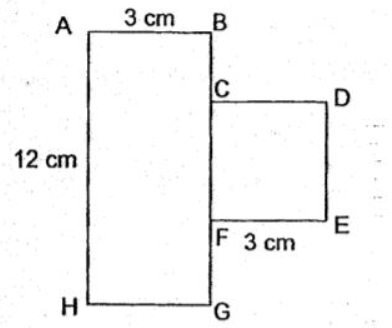Question 4. The total mass of two 2 textbooks and 7 dictionaries is 1735g. The total mass of 2 textbooks and 1 dictionary is 385 g. What is the mass of 1 dictionary? *
2 points
Question 5. Miss Allana wants to buy 28 boxes of pencils. What is the least amount of money she has to pay? *
2 points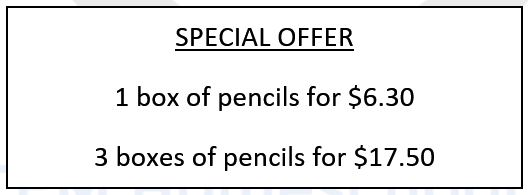Question 6. Jared bought 2 pens and 2 erasers for \$7.80 from the bookstore. Each pen cost \$0.90 more than each eraser. What is the cost of an eraser? *
3 points
Question 7. Mrs. Bell bought some sweets for her students. If she gave 6 sweets to each student, she would have 5 sweets left. If she gave 8 sweets to each student, she would need 3 more sweets. How many sweets did she buy? *
3 points
Question 8. Sofia spent 4/10 of her money on a handbag and 5/10 of it on a pair of shoes. She has \$36 left. How much money did she have at first? *
2 points
Question 9. Anna has 96 beads. She gave 1/3 of it to Mary and 1/4 of it to Alice. How many beads does she have left? *
2 points
Question 10. The figure below shows a big, square field of side 25m. Four square tiles of side 4m are placed on all four corners of the field. Find the area of the field that is not covered by tiles. *
3 pointsQuestion 11. Penny and Holly saved \$95 altogether. Penny and Bruce saved \$205 altogether. Bruce saved three times as much as Holly. How many did the 3 children save altogether? *
4 points
Question 12. Chester saved \$17.50 in 5 days. Each day, he saved \$.50 more than the day before. How much did he save on the first day? *
3 points
Question 13. The sum of two numbers is 40.59. The larger number is 14.45 greater than the smaller number. What is the larger number? *
3 points
Question 14. A farmer bought 1kg of soil. He used 2/3 kg of it for his tomatoes plants and 1/4 kg of it for his pepper plant. What fraction of soil does he have left? *
2 points
Question 15. A big box contains 3 times as many apples as a small box. If 1 big box and 2 small boxes contain 30 apples, how many apples does the big box contain? *
3 points
Question 16. A plane left Trinidad at 2205 on Monday and arrived at Miami at 0215 on Tuesday. How long was the flight from Trinidad to Miami? Give your answer in hours and minutes. *
2 points
Question 17. Carrie is facing the zoo. She makes a 1/4 turn anticlockwise. She then makes a 270 degrees turn clockwise. Where is she facing now? *
2 points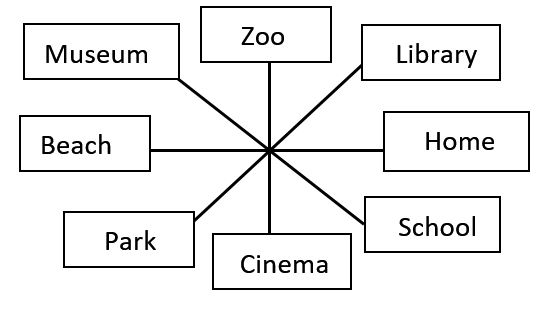Question 18. The figure below shows a square and a rectangle. The area of the square is 16cm^2. The breadth of the rectangle is the same as the length of the square. The length of the rectangle is three times its breadth. Find the perimeter of the rectangle. *
2 points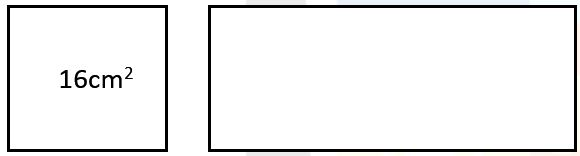Question 19. Tyler, Luke and Leia had a total of 640 toy cars. Tyler had 68 more toy cars than Luke. Leia had twice as many toy cars as Tyler. How many toy cars does Luke have? *
4 points
Question 20. Square X and rectangle Y have the same area. Find the perimeter of rectangle Y. *
2 points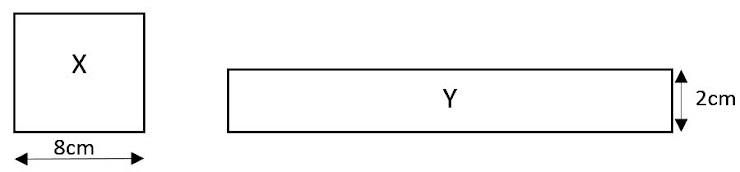A copy of your responses will be emailed to the address you provided.